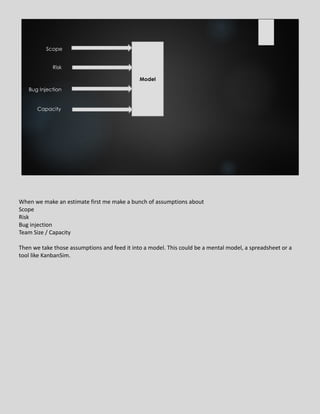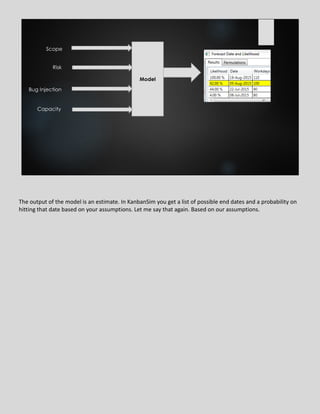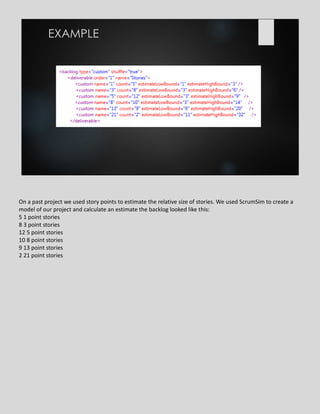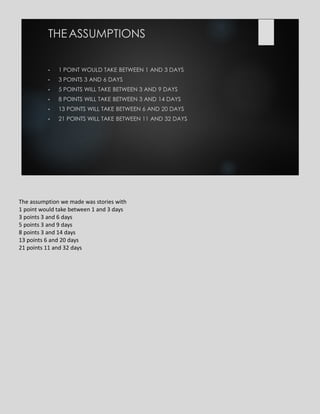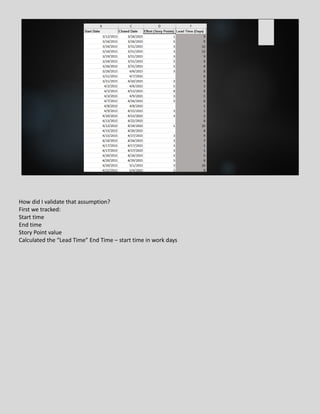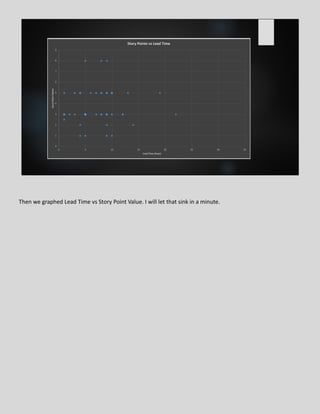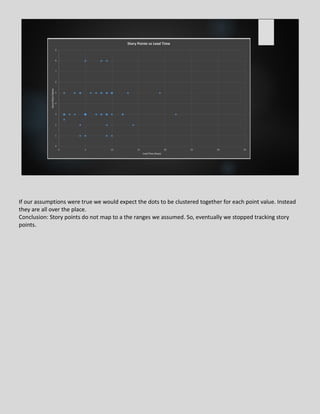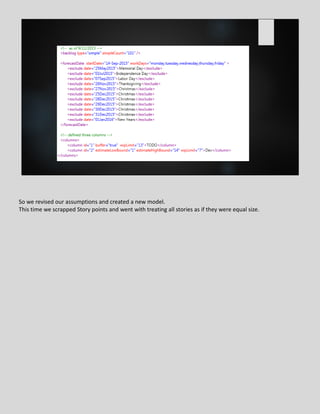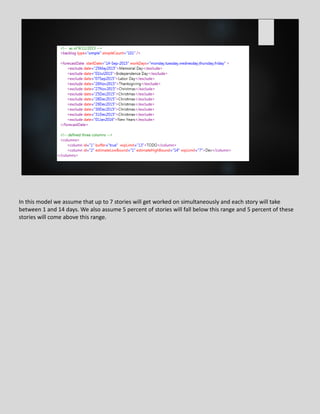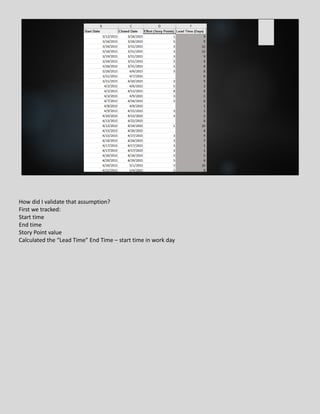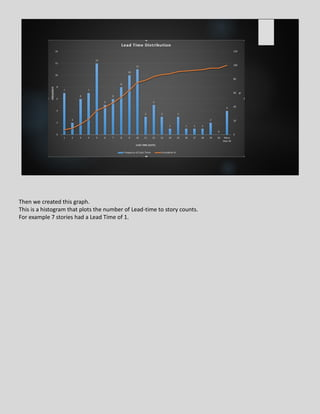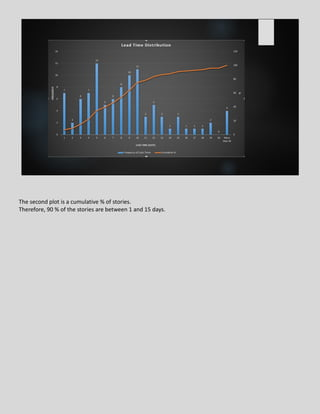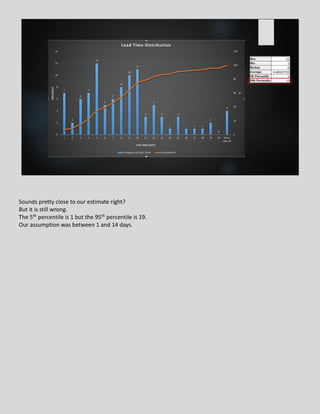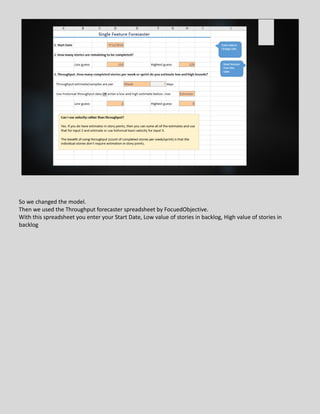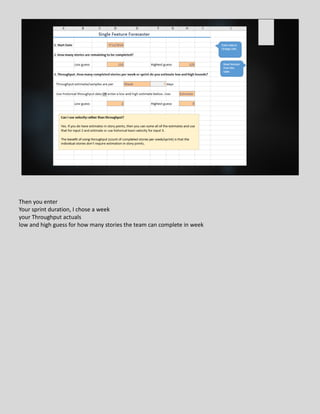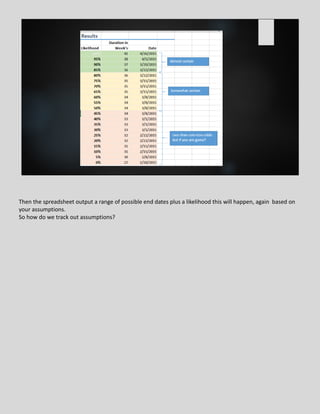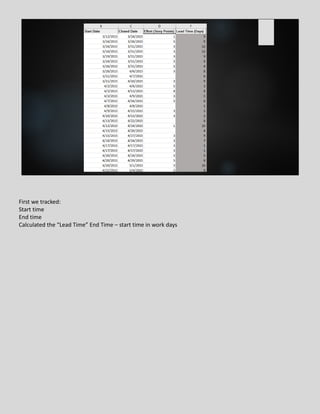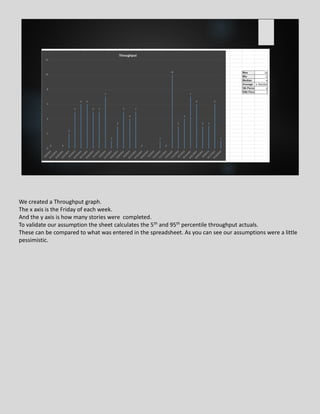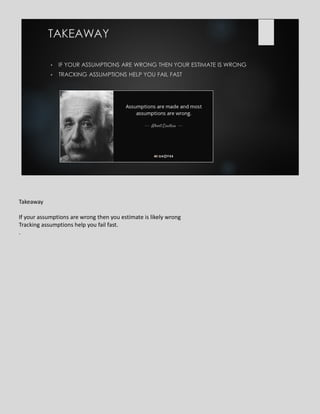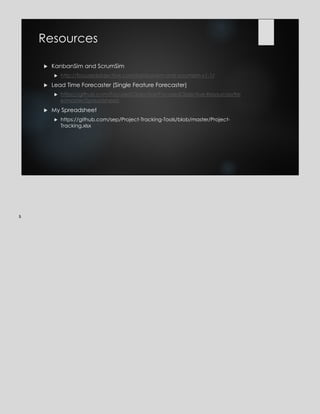Upcoming SlideShareSEJ Summit 2015: The Pareto Principle 2.0: Using Analytics to Find the Right ...
1 of 23

1. Round Tripping Your Assumptions ‘CAUSE YOU KNOW WHAT IT MEANS TO ASSUME…
2. Model Scope Risk Bug Injection Capacity When we make an estimate first me make a bunch of assumptions about Scope Risk Bug injection Team Size / Capacity Then we take those assumptions and feed it into a model. This could be a mental model, a spreadsheet or a tool like KanbanSim.
3. Model Scope Risk Bug Injection Capacity Estimate The output of the model is an estimate. In KanbanSim you get a list of possible end dates and a probability on hitting that date based on your assumptions. Let me say that again. Based on our assumptions.
4. You know what they say about what means to assume, right. You look like this guy Do how do we prevent looking like this? How will we know when our estimate is off? In the past, I knew my estimate was wrong when the due date went whizzing past me
5. There is a better way. By tracking your assumptions you can tell your estimate is off when your assumptions turn out to be not true. Then you can discuss your assumptions with your stakeholders instead of haggling over end dates and dollar amounts
6. EXAMPLE On a past project we used story points to estimate the relative size of stories. We used ScrumSim to create a model of our project and calculate an estimate the backlog looked like this: 5 1 point stories 8 3 point stories 12 5 point stories 10 8 point stories 9 13 point stories 2 21 point stories
7. • 1 POINT WOULD TAKE BETWEEN 1 AND 3 DAYS • 3 POINTS 3 AND 6 DAYS • 5 POINTS WILL TAKE BETWEEN 3 AND 9 DAYS • 8 POINTS WILL TAKE BETWEEN 3 AND 14 DAYS • 13 POINTS WILL TAKE BETWEEN 6 AND 20 DAYS • 21 POINTS WILL TAKE BETWEEN 11 AND 32 DAYS THEASSUMPTIONS The assumption we made was stories with 1 point would take between 1 and 3 days 3 points 3 and 6 days 5 points 3 and 9 days 8 points 3 and 14 days 13 points 6 and 20 days 21 points 11 and 32 days
8. How did I validate that assumption? First we tracked: Start time End time Story Point value Calculated the “Lead Time” End Time – start time in work days
9. Then we graphed Lead Time vs Story Point Value. I will let that sink in a minute.
10. If our assumptions were true we would expect the dots to be clustered together for each point value. Instead they are all over the place. Conclusion: Story points do not map to a the ranges we assumed. So, eventually we stopped tracking story points.
11. So we revised our assumptions and created a new model. This time we scrapped Story points and went with treating all stories as if they were equal size.
12. In this model we assume that up to 7 stories will get worked on simultaneously and each story will take between 1 and 14 days. We also assume 5 percent of stories will fall below this range and 5 percent of these stories will come above this range.
13. How did I validate that assumption? First we tracked: Start time End time Story Point value Calculated the “Lead Time” End Time – start time in work day
14. Then we created this graph. This is a histogram that plots the number of Lead-time to story counts. For example 7 stories had a Lead Time of 1.
15. The second plot is a cumulative % of stories. Therefore, 90 % of the stories are between 1 and 15 days.
16. Sounds pretty close to our estimate right? But it is still wrong. The 5th percentile is 1 but the 95th percentile is 19. Our assumption was between 1 and 14 days.
17. So we changed the model. Then we used the Throughput forecaster spreadsheet by FocuedObjective. With this spreadsheet you enter your Start Date, Low value of stories in backlog, High value of stories in backlog
18. Then you enter Your sprint duration, I chose a week your Throughput actuals low and high guess for how many stories the team can complete in week
19. Then the spreadsheet output a range of possible end dates plus a likelihood this will happen, again based on your assumptions. So how do we track out assumptions?
20. First we tracked: Start time End time Calculated the “Lead Time” End Time – start time in work days
21. We created a Throughput graph. The x axis is the Friday of each week. And the y axis is how many stories were completed. To validate our assumption the sheet calculates the 5th and 95th percentile throughput actuals. These can be compared to what was entered in the spreadsheet. As you can see our assumptions were a little pessimistic.
22. • IF YOUR ASSUMPTIONS ARE WRONG THEN YOUR ESTIMATE IS WRONG • TRACKING ASSUMPTIONS HELP YOU FAIL FAST TAKEAWAY Takeaway If your assumptions are wrong then you estimate is likely wrong Tracking assumptions help you fail fast. .
23. Resources  KanbanSim and ScrumSim  http://focusedobjective.com/kanbansim-and-scrumsim-v1-1/  Lead Time Forecaster (Single Feature Forecaster)  https://github.com/FocusedObjective/FocusedObjective.Resources/tre e/master/Spreadsheets  My Spreadsheet  https://github.com/sep/Project-Tracking-Tools/blob/master/Project- Tracking.xlsx s